# 【数据结构】 常见的八大排序算法（下）

+关注继续查看

### 2.2 挖坑法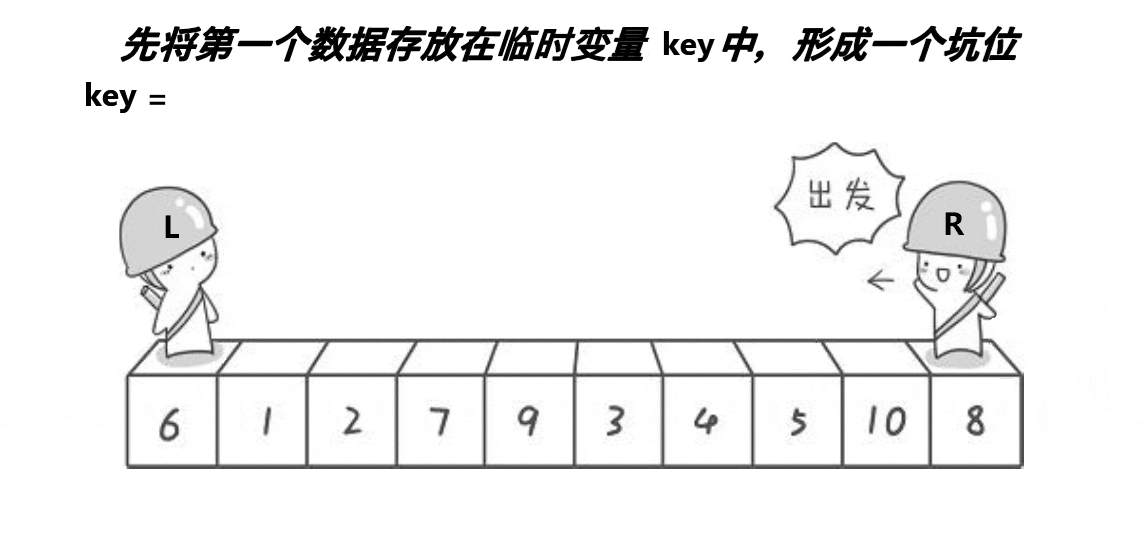int QuickSort2(int* arr, int left, int right) //快速排序挖坑法
{
int mid = GetMidIndex(arr, left, right); //三数取中
Swap(&arr[left], &arr[mid]);使中间值永远在最左，便于决定谁先走
int hole = left; //对key值保存
int key = arr[left];//保存坑位下标
while (left < right)
{
while (left < right && arr[right] >= key) //右边找小
{
--right;
}
arr[hole] = arr[right]; //填坑
hole = right;
while (left < right && arr[left] <= key) //左边找大
{
++left;
}
arr[hole] = arr[left];//填坑
hole = left;
}
arr[hole] = key; //相遇时
return hole;
}

### 2.3 前后指针法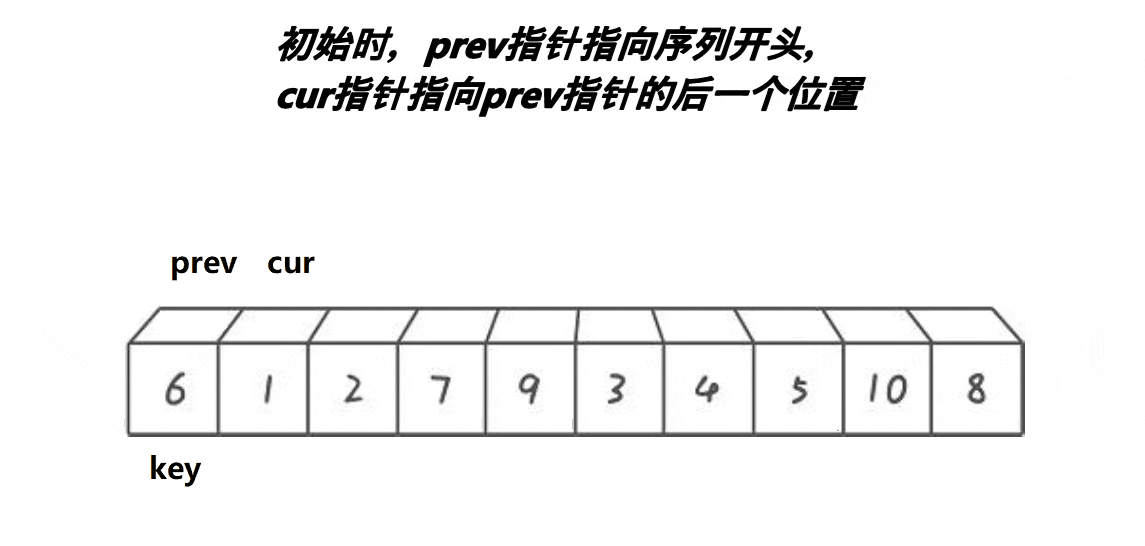int QuickSort3(int* arr, int left, int right) //前后指针版
{
int mid = GetMidIndex(arr, left, right);
Swap(&arr[left], &arr[mid]);
int key = left;
int prev = left, cur = left + 1; //初始化前后指针
while (cur <= right)
{
if (arr[cur] < arr[key] && ++prev != cur)//逻辑与防止交换同一个位置的元素
{
Swap(&arr[cur], &arr[++prev]);
}
cur++;
}
Swap(&arr[key], &arr[prev]);//遍历结束将key值放在定位点
return prev;
}

++prev!=cur 是对防止同一位置元素进行交换的简单优化，实际中，前后指针法推荐掌握，简单理解易于操控。

void _QuickSort3(int* arr, int begin, int end) //前后指针法优化：三指针法
{
if (begin >= end)
{
return;
}
if ((end - begin + 1) < 15)
{
InsertSort(arr + begin, end - begin + 1);
}
else
{
int mid = GetMidIndex(arr, begin, end);
Swap(&arr[begin], &arr[mid]);

int key = arr[begin];
int left = begin, right = end, cur = begin + 1;
while (cur <= right)
{
if (arr[cur] < key)
{
Swap(&arr[left], &arr[cur]);
left++;
cur++;
}
else if (arr[cur] > key)
{
Swap(&arr[cur], &arr[right]);
right--;
}
else//arr[cur]==key时
{
cur++;
}
}
QuickSortPlus(arr, begin, left - 1);
QuickSortPlus(arr, right + 1, end);
}

}

### 2.4 快排非递归版

void QuickSortNonR(int* arr, int begin, int end) // 快速排序 非递归实现
{
ST st; //首先构建一个栈
StackInit(&st);
StackPush(&st, begin); //将左右区间入栈
StackPush(&st, end);

while (!StackEmpty(&st))
{
int right = StackTop(&st);
StackPop(&st);
int left = StackTop(&st);
StackPop(&st);

int key = QuickSort3(arr, left, right); //排序：利用上面的前后指针法进行排序

// [begin,key-1]  [key+1,end]

if (key + 1 < right)//key+1==right说明区间只剩一个值时，则可以认为有序
{
StackPush(&st, key + 1);
StackPush(&st, right);
}

if (left < key - 1)
{
StackPush(&st, left);
StackPush(&st, key - 1);
}

}
StackDestroy(&st); //栈销毁
}

# 四、归并排序

## 1、归并排序递归版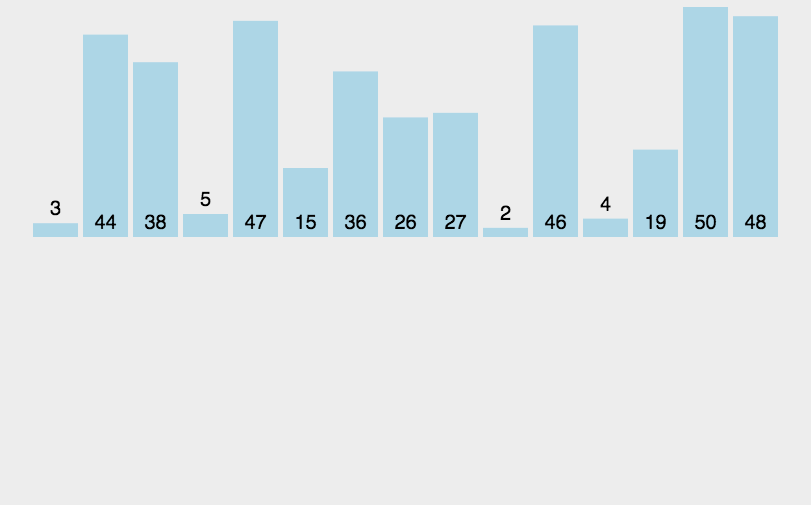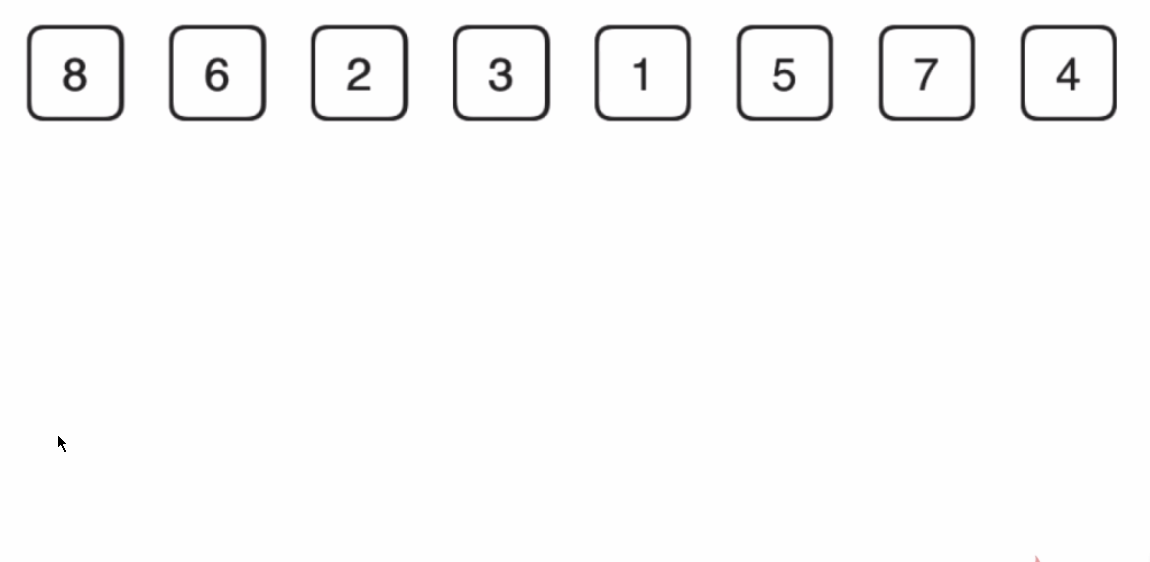void _MergeSort(int* arr, int begin, int end, int* tmp) //归并排序
{
if (begin >= end) //只有一个元素或没有元素为有序
{
return;
}
int mid = (begin + end) / 2;
_MergeSort(arr, begin, mid, tmp);
_MergeSort(arr, mid + 1, end, tmp);
//左区间和右区间有序后开始归并
int i = begin;
int begin1 = begin, end1 = mid;
int begin2 = mid + 1, end2 = end;
while (begin1 <= end1 && begin2 <= end2)
{
if (arr[begin1] <= arr[begin2])//升序
{
tmp[i++] = arr[begin1++];
}
else
{
tmp[i++] = arr[begin2++];
}
}
while (begin1 <= end1)
{
tmp[i++] = arr[begin1++];
}
while (begin2 <= end2)
{
tmp[i++] = arr[begin2++];
}
memcpy(arr + begin, tmp + begin, sizeof(int) * (end - begin + 1));//拷贝回数组arr
}
void MergeSort(int* arr, int begin, int end)
{
int* tmp = (int*)malloc(sizeof(int) * (end - begin));//创建暂存数据数组，以保存归并好的数据
_MergeSort(arr, begin, end - 1, tmp);

free(tmp);//释放
tmp = NULL;
}

## 2、递归排序非递归版

void MergeSortNonR(int* a, int n) //归并排序非递归版
{
int* tmp = (int*)malloc(sizeof(int) * n);
if (tmp == NULL)
{
perror("malloc fail");
exit(-1);
}
int gap = 1;//定义数组归并距离
//（初始gap为1即每个数组只有一个元素，此时每个数组都为有序数组）
while (gap < n)//归并趟次
{
for (int i = 0; i < n; i += gap * 2)//分组归并
{
//划分区间
int begin1 = i, end1 = i + gap - 1;
int begin2 = i + gap, end2 = i + 2 * gap - 1;
//判断越界的情况
//这种情况不用考虑归并（已经有序）
if (end1 >= n || begin2 >= n)
{
break;
}
//这种情况需要归并
if (end2 >= n)
{
end2 = n - 1;
}
//归并
int p = i;
while (begin1 <= end1 && begin2 <= end2)
{
if (a[begin1] < a[begin2])
{
tmp[p++] = a[begin1++];
}
else
{
tmp[p++] = a[begin2++];
}
}
while (begin1 <= end1)
{
tmp[p++] = a[begin1++];
}
while (begin2 <= end2)
{
tmp[p++] = a[begin2++];
}
//拷贝排序后数据到原数组
for (int j = i; j <= end2; j++)
{
a[j] = tmp[j];
}
}
gap *= 2;
}
free(tmp);//释放
tmp = NULL;


# 五、计数排序 - 非比较排序

统计相同元素出现次数：在排序数组中找到最大最小的数据，算出对应范围（max - min +1）并创建对应长度个数组用来计数（相对位置遍历一次+1），遍历排序数组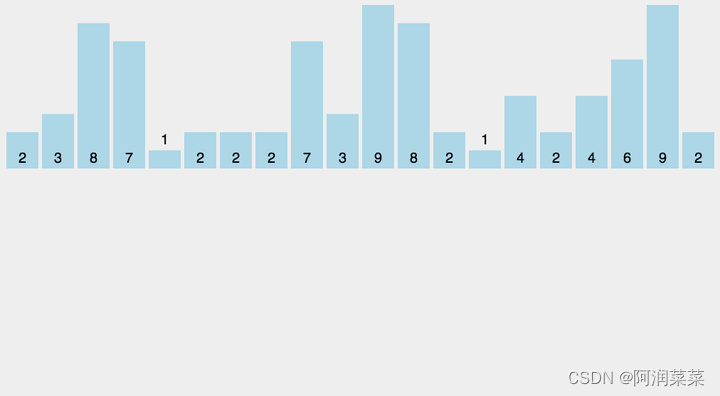代码实现：

void CountSort(int* a, int n) //计数排序
{
//遍历找出数组最大最小值（算出范围）
int max = a, min = a;
for (int i = 1; i < n; i++)
{
if (a[i] > max)
max = a[i];
if (a[i] < min)
min = a[i];
}
int range = max - min + 1;
//开辟对应长度个计数数组
int* count = (int*)malloc(sizeof(int) * range);
if (count == NULL)
{
perror("malloc fail");
exit(-1);
}
//初始化数组计数为0
memset(count, 0, sizeof(int) * range);
//遍历计数据出现次数
for (int i = 0; i < n; i++)
{
count[a[i] - min]++;
//a[i] - min：数据与下标构成的相对映射关系
}
//排入原数组
int p = 0;
for (int i = 0; i < range; i++)
{
while (count[i]--)
{
a[p++] = i + min;
}
}
free(count);//释放内存
count = NULL;
}

# 六、对排序的分析总结

## 排序算法复杂度及稳定性总结：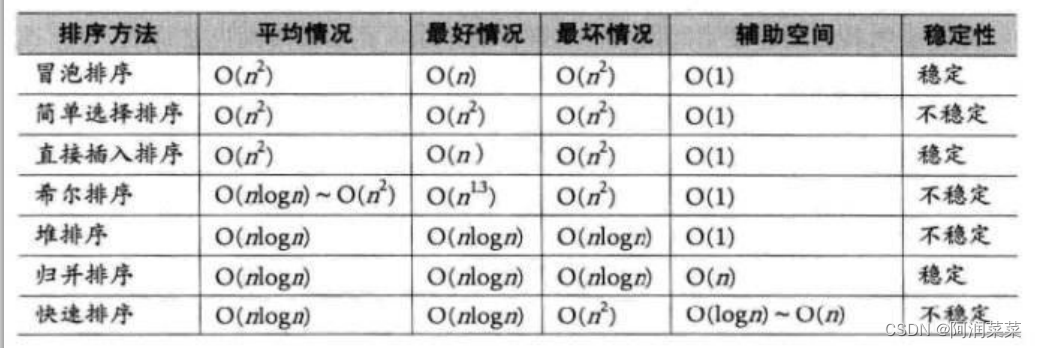思维导图：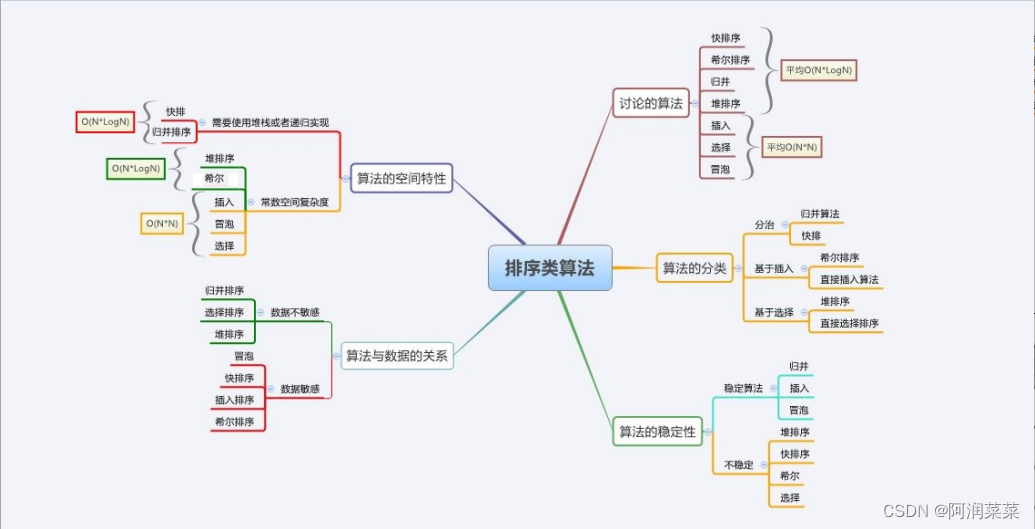💙欢迎大家批评指正|
1月前
|

【数据结构】排序算法大总结

93 3
|
3月前
|

31 0
|
3月前
|

【数据结构】 常见的八大排序算法（上）
【数据结构】 常见的八大排序算法（上）
155 1
|
4月前
|

165 0